# Statistics review 14: Logistic regression

## Abstract

This review introduces logistic regression, which is a method for modelling the dependence of a binary response variable on one or more explanatory variables. Continuous and categorical explanatory variables are considered.

## Introduction

Logistic regression provides a method for modelling a binary response variable, which takes values 1 and 0. For example, we may wish to investigate how death (1) or survival (0) of patients can be predicted by the level of one or more metabolic markers. As an illustrative example, consider a sample of 2000 patients whose levels of a metabolic marker have been measured. Table 1 shows the data grouped into categories according to metabolic marker level, and the proportion of deaths in each category is given. The proportions of deaths are estimates of the probabilities of death in each category. Figure 1 shows a plot of these proportions. It suggests that the probability of death increases with the metabolic marker level. However, it can be seen that the relationship is nonlinear and that the probability of death changes very little at the high or low extremes of marker level. This pattern is typical because proportions cannot lie outside the range from 0 to 1. The relationship can be described as following an 'S'-shaped curve.

## Logistic regression with a single quantitative explanatory variable

The logistic or logit function is used to transform an 'S'-shaped curve into an approximately straight line and to change the range of the proportion from 0–1 to -∞ to +∞.

The logit function is defined as the natural logarithm (ln) of the odds  of death. That is,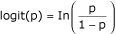Where p is the probability of death.

Figure 2 shows the logit-transformed proportions from Fig. 1. The points now follow an approximately straight line. The relationship between probability of death and marker level x could therefore be modelled as follows:

logit(p) = a + bx

Although this model looks similar to a simple linear regression model, the underlying distribution is binomial and the parameters a and b cannot be estimated in exactly the same way as for simple linear regression. Instead, the parameters are usually estimated using the method of maximum likelihood, which is discussed below.

## Binomial distribution

When the response variable is binary (e.g. death or survival), then the probability distribution of the number of deaths in a sample of a particular size, for given values of the explanatory variables, is usually assumed to be binomial. The probability that the number of deaths in a sample of size n is exactly equal to a value r is given by nCrpr(1 - p)n - r, where nCr = n!/(r!(n - r)!) is the number of ways r individuals can be chosen from n and p is the probability of an individual dying. (The probability of survival is 1 - p.)

For example, using the first row of the data in Table 1, the probability that seven deaths occurred out of 182 patients is given by 182C7p7(1 - p)175. If the probability of death is assumed to be 0.04, then the probability that seven deaths occurred is 182C7 × 0.047 × 0.86175 = 0.152. This probability, calculated on the assumption of a binomial distribution with parameter p = 0.04, is called a likelihood.

### Maximum likelihood estimation

Maximum likelihood estimation involves finding the value(s) of the parameter(s) that give rise to the maximum likelihood. For example, again we shall take the seven deaths occurring out of 182 patients and use maximum likelihood estimation to estimate the probability of death, p. Figure 3 shows the likelihood calculated for a range of values of p. From the graph it can be seen that the value of p giving the maximum likelihood is close to 0.04. This value is the maximum likelihood estimate (MLE) of p. Mathematically, it can be shown that the MLE in this case is 7/182.

In more complicated situations, iterative techniques are required to find the maximum likelihood and the associated parameter values, and a computer package is required.

### Odds

The model logit(p) = a + bx is equivalent to the following: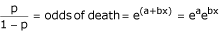or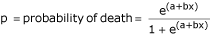Because the explanatory variable x increases by one unit from x to x + 1, the odds of death change from eaebx to eaeb(x + 1) = eaebxeb. The odds ratio (OR) is therefore eaebxeb/eaebx = eb. The odds ratio eb has a simpler interpretation in the case of a categorical explanatory variable with two categories; in this case it is just the odds ratio for one category compared with the other.

Estimates of the parameters a and b are usually obtained using a statistical package, and the output for the data summarized in Table 1 is given in Table 2. From the output, b = 1.690 and eb OR = 5.4. This indicates that, for example, the odds of death for a patient with a marker level of 3.0 is 5.4 times that of a patient with marker level 2.0.

### Predicted probabilities

The model can be used to calculate the predicted probability of death (p) for a given value of the metabolic marker. For example, patients with metabolic marker level 2.0 and 3.0 have the following respective predicted probabilities of death: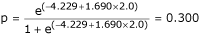andThe corresponding odds of death for these patients are 0.300/(1 - 0.300) = 0.428 and 0.700/(1 - 0.700) = 2.320, giving an odds ratio of 2.320/0.428 = 5.421, as above.

The metabolic marker level at which the predicted probability equals 0.5 – that is, at which the two possible outcomes are equally likely – is called the median effective level (EL50). Solving the equation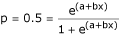gives x = EL50 = a/b

For the example data, EL50 = 4.229/1.690 = 2.50, indicating that at this marker level death or survival are equally likely.

## Assessment of the fitted model

After estimating the coefficients, there are several steps involved in assessing the appropriateness, adequacy and usefulness of the model. First, the importance of each of the explanatory variables is assessed by carrying out statistical tests of the significance of the coefficients. The overall goodness of fit of the model is then tested. Additionally, the ability of the model to discriminate between the two groups defined by the response variable is evaluated. Finally, if possible, the model is validated by checking the goodness of fit and discrimination on a different set of data from that which was used to develop the model.

### Tests and confidence intervals for the parameters

#### The Wald statistic

Wald χ2 statistics are used to test the significance of individual coefficients in the model and are calculated as follows: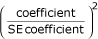Each Wald statistic is compared with a χ2 distribution with 1 degree of freedom. Wald statistics are easy to calculate but their reliability is questionable, particularly for small samples. For data that produce large estimates of the coefficient, the standard error is often inflated, resulting in a lower Wald statistic, and therefore the explanatory variable may be incorrectly assumed to be unimportant in the model. Likelihood ratio tests (see below) are generally considered to be superior.

The Wald tests for the example data are given in Table 2. The test for the coefficient of the metabolic marker indicates that the metabolic marker contributes significantly in predicting death.

The constant has no simple practical interpretation but is generally retained in the model irrespective of its significance.

#### Likelihood ratio test

The likelihood ratio test for a particular parameter compares the likelihood of obtaining the data when the parameter is zero (L0) with the likelihood (L1) of obtaining the data evaluated at the MLE of the parameter. The test statistic is calculated as follows:

-2 × ln(likelihood ratio) = -2 × ln(L0/L1) = -2 × (lnL0 - lnL1)

It is compared with a χ2 distribution with 1 degree of freedom. Table 3 shows the likelihood ratio test for the example data obtained from a statistical package and again indicates that the metabolic marker contributes significantly in predicting death.

### Goodness of fit of the model

The goodness of fit or calibration of a model measures how well the model describes the response variable. Assessing goodness of fit involves investigating how close values predicted by the model are to the observed values.

When there is only one explanatory variable, as for the example data, it is possible to examine the goodness of fit of the model by grouping the explanatory variable into categories and comparing the observed and expected counts in the categories. For example, for each of the 182 patients with metabolic marker level less than one the predicted probability of death was calculated using the formula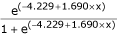where x is the metabolic marker level for an individual patient. This gives 182 predicted probabilities from which the arithmetic mean was calculated, giving a value of 0.04. This was repeated for all metabolic marker level categories. Table 4 shows the predicted probabilities of death in each category and also the expected number of deaths calculated as the predicted probability multiplied by the number of patients in the category. The observed and the expected numbers of deaths can be compared using a χ2 goodness of fit test, providing the expected number in any category is not less than 5. The null hypothesis for the test is that the numbers of deaths follow the logistic regression model. The χ2 test statistic is given by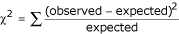The test statistic is compared with a χ2 distribution where the degrees of freedom are equal to the number of categories minus the number of parameters in the logistic regression model. For the example data the χ2 statistic is 2.68 with 9 - 2 = 7 degrees of freedom, giving P = 0.91, suggesting that the numbers of deaths are not significantly different from those predicted by the model.

### The Hosmer–Lemeshow test

The Hosmer–Lemeshow test is a commonly used test for assessing the goodness of fit of a model and allows for any number of explanatory variables, which may be continuous or categorical. The test is similar to a χ2 goodness of fit test and has the advantage of partitioning the observations into groups of approximately equal size, and therefore there are less likely to be groups with very low observed and expected frequencies. The observations are grouped into deciles based on the predicted probabilities. The test statistic is calculated as above using the observed and expected counts for both the deaths and survivals, and has an approximate χ2 distribution with 8 (=10 - 2) degrees of freedom. Calibration results for the model from the example data are shown in Table 5. The Hosmer–Lemeshow test (P = 0.576) indicates that the numbers of deaths are not significantly different from those predicted by the model and that the overall model fit is good.

Further checks can be carried out on the fit for individual observations by inspection of various types of residuals (differences between observed and fitted values). These can identify whether any observations are outliers or have a strong influence on the fitted model. For further details see, for example, Hosmer and Lemeshow .

### R2 for logistic regression

Most statistical packages provide further statistics that may be used to measure the usefulness of the model and that are similar to the coefficient of determination (R2) in linear regression . The Cox & Snell and the Nagelkerke R2 are two such statistics. The values for the example data are 0.44 and 0.59, respectively. The maximum value that the Cox & Snell R2 attains is less than 1. The Nagelkerke R2 is an adjusted version of the Cox & Snell R2 and covers the full range from 0 to 1, and therefore it is often preferred. The R2 statistics do not measure the goodness of fit of the model but indicate how useful the explanatory variables are in predicting the response variable and can be referred to as measures of effect size. The value of 0.59 indicates that the model is useful in predicting death.

### Discrimination

The discrimination of a model – that is, how well the model distinguishes patients who survive from those who die – can be assessed using the area under the receiver operating characteristic curve (AUROC) . The value of the AUROC is the probability that a patient who died had a higher predicted probability than did a patient who survived. Using a statistical package to calculate the AUROC for the example data gave a value of 0.90 (95% C.I. 0.89 to 0.91), indicating that the model discriminates well.

### Validation

When the goodness of fit and discrimination of a model are tested using the data on which the model was developed, they are likely to be over-estimated. If possible, the validity of model should be assessed by carrying out tests of goodness of fit and discrimination on a different data set from the original one.

## Logistic regression with more than one explanatory variable

We may wish to investigate how death or survival of patients can be predicted by more than one explanatory variable. As an example, we shall use data obtained from patients attending an accident and emergency unit. Serum metabolite levels were investigated as potentially useful markers in the early identification of those patients at risk for death. Two of the metabolic markers recorded were lactate and urea. Patients were also divided into two age groups: <70 years and ≥70 years.

Like ordinary regression, logistic regression can be extended to incorporate more than one explanatory variable, which may be either quantitative or qualitative. The logistic regression model can then be written as follows:

logit(p) = a + b1x1 + b2x2 + ... + bixi

where p is the probability of death and x1, x2 ... xi are the explanatory variables.

The method of including variables in the model can be carried out in a stepwise manner going forward or backward, testing for the significance of inclusion or elimination of the variable at each stage. The tests are based on the change in likelihood resulting from including or excluding the variable . Backward stepwise elimination was used in the logistic regression of death/survival on lactate, urea and age group. The first model fitted included all three variables and the tests for the removal of the variables were all significant as shown in Table 6.

Therefore all the variables were retained. For these data, forward stepwise inclusion of the variables resulted in the same model, though this may not always be the case because of correlations between the explanatory variables. Several models may produce equally good statistical fits for a set of data and it is therefore important when choosing a model to take account of biological or clinical considerations and not depend solely on statistical results.

The output from a statistical package is given in Table 7. The Wald tests also show that all three explanatory variables contribute significantly to the model. This is also seen in the confidence intervals for the odds ratios, none of which include 1 .

From Table 7 the fitted model is:

logit(p) = -5.716 + (0.270 × lactate) + (0.053 × urea) + (1.425 × age group)

Because there is more than one explanatory variable in the model, the interpretation of the odds ratio for one variable depends on the values of other variables being fixed. The interpretation of the odds ratio for age group is relatively simple because there are only two age groups; the odds ratio of 4.16 indicates that, for given levels of lactate and urea, the odds of death for patients in the ≥70 years group is 4.16 times that in the <70 years group. The odds ratio for the quantitative variable lactate is 1.31. This indicates that, for a given age group and level of urea, for an increase of 1 mmol/l in lactate the odds of death are multiplied by 1.31. Similarly, for a given age group and level of lactate, for an increase of 1 mmol/l in urea the odds of death are multiplied by 1.05.

The Hosmer–Lemeshow test results (χ2 = 7.325, 8 degrees of freedom, P = 0.502) indicate that the goodness of fit is satisfactory. However, the Nagelkerke R2 value was 0.17, suggesting that the model is not very useful in predicting death. Although the contribution of the three explanatory variables in the prediction of death is statistically significant, the effect size is small.

The AUROC for these data gave a value of 0.76 ((95% C.I. 0.69 to 0.82)), indicating that the discrimination of the model is only fair.

## Assumptions and limitations

The logistic transformation of the binomial probabilities is not the only transformation available, but it is the easiest to interpret, and other transformations generally give similar results.

In logistic regression no assumptions are made about the distributions of the explanatory variables. However, the explanatory variables should not be highly correlated with one another because this could cause problems with estimation.

Large sample sizes are required for logistic regression to provide sufficient numbers in both categories of the response variable. The more explanatory variables, the larger the sample size required. With small sample sizes, the Hosmer–Lemeshow test has low power and is unlikely to detect subtle deviations from the logistic model. Hosmer and Lemeshow recommend sample sizes greater than 400.

The choice of model should always depend on biological or clinical considerations in addition to statistical results.

## Conclusion

Logistic regression provides a useful means for modelling the dependence of a binary response variable on one or more explanatory variables, where the latter can be either categorical or continuous. The fit of the resulting model can be assessed using a number of methods.

## Abbreviations

AUROC:

= area under the receiver operating characteristic curve

C.I.:

= confidence interval

ln:

= natural logarithm

logit:

= natural logarithm of the odds

MLE:

= maximum likelihood estimate

OR:

= odds ratio

ROC:

= receiver operating characteristic curve.

## References

1. Kirkwood BR, Sterne JAC: Essential Medical Statistics. 2nd edition. Oxford, UK: Blackwell Science Ltd; 2003.

2. Hosmer DW, Lemeshow S: Applied Logistic Regression. 2nd edition. New York, USA: John Wiley and Sons; 2000.

3. Bewick V, Cheek L, Ball J: Statistics review 7: Correlation and regression. Crit Care 2003, 7: 451-459. 10.1186/cc2401

4. Bewick V, Cheek L, Ball J: Statistics review 13: Receiver operating characteristic (ROC) curves. Crit Care 2004, 8: 508-512. 10.1186/cc3000

5. Bewick V, Cheek L, Ball J: Statistics review 11: Assessing risk. Crit Care 2004, 8: 287-291. 10.1186/cc2908

## Author information

Authors

### Corresponding author

Correspondence to Viv Bewick.

### Competing interests

The author(s) declare that they have no competing interests.

## Authors’ original submitted files for images

Below are the links to the authors’ original submitted files for images.

## Rights and permissions

Reprints and Permissions

Bewick, V., Cheek, L. & Ball, J. Statistics review 14: Logistic regression. Crit Care 9, 112 (2005). https://doi.org/10.1186/cc3045

• Published:

• DOI: https://doi.org/10.1186/cc3045

### Keywords

• binomial distribution
• Hosmer–Lemeshow test
• likelihood
• likelihood ratio test
• logit function
• maximum likelihood estimation
• median effective level
• odds
• odds ratio
• predicted probability
• Wald test